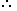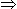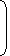# Tips to solve problems related to Chain Rule1. Direct Proportion:Two quantities are said to be directly proportional, if on the increase (or decrease) of the one, the other increases (or decreases) to the same extent.Eg. Cost is directly proportional to the number of articles.
(More Articles, More Cost)
2. Indirect Proportion:Two quantities are said to be indirectly proportional, if on the increase of the one, the orther decreases to the same extent and vice-versa.Eg. The time taken by a car is covering a certain distance is inversely proportional to the speed of the car. (More speed, Less is the time taken to cover a distance.)Note: In solving problems by chain rule, we compare every item with the term to be found out
1. 3 pumps, working 8 hours a day, can empty a tank in 2 days. How many hours a day must 4 pumps work to empty the tank in 1 day?
 A. 9 B. 10 C. 11 D. 12

Explanation:

Let the required number of working hours per day be x.

More pumps, Less working hours per day (Indirect Proportion)

Less days, More working hours per day (Indirect Proportion)

 Pumps 4 : 3:: 8 : x Days 1 : 24 x 1 x x = 3 x 2 x 8x = (3 x 2 x 8)/ (4)x = 12.

2. If the cost of x metres of wire is d rupees, then what is the cost of y metres of wire at the same rate?
A.
 Rs.xyd
B. Rs. (xd)
C. Rs. (yd)
D.
 Rs.ydx

Explanation:

Cost of x metres = Rs. d.

 Cost of 1 metre = Rs.dx
 Cost of y metres = Rs.d . y= Rs.yd. x x

#### Running at the same constant rate, 6 identical machines can produce a total of 270 bottles per minute. At this rate, how many bottles could 10 such machines produce in 4 minutes?

Correct! Wrong!

#### 39 persons can repair a road in 12 days, working 5 hours a day. In how many days will 30 persons, working 6 hours a day, complete the work?

Correct! Wrong!

#### If a quarter kg of potato costs 60 paise, how many paise will 200 gm cost?

Correct! Wrong!

#### A wheel that has 6 cogs is meshed with a larger wheel of 14 cogs. When the smaller wheel has made 21 revolutions, then the number of revolutions mad by the larger wheel is:

Correct! Wrong!

#### If 7 spiders make 7 webs in 7 days, then 1 spider will make 1 web in how many days?

Correct! Wrong!

QUIZ ON CHAIN RULE RELATED PROBLEMS IN APTITUDE SECTION
GREAT JOB!!!!